Simplification And Approximation - Practice Test

# Simplification And Approximation - Practice Test

Test Description

## 5 Questions MCQ Test UPSC Prelims Paper 2 CSAT - Quant, Verbal & Decision Making | Simplification And Approximation - Practice Test

Simplification And Approximation - Practice Test for Quant 2023 is part of UPSC Prelims Paper 2 CSAT - Quant, Verbal & Decision Making preparation. The Simplification And Approximation - Practice Test questions and answers have been prepared according to the Quant exam syllabus.The Simplification And Approximation - Practice Test MCQs are made for Quant 2023 Exam. Find important definitions, questions, notes, meanings, examples, exercises, MCQs and online tests for Simplification And Approximation - Practice Test below.
Solutions of Simplification And Approximation - Practice Test questions in English are available as part of our UPSC Prelims Paper 2 CSAT - Quant, Verbal & Decision Making for Quant & Simplification And Approximation - Practice Test solutions in Hindi for UPSC Prelims Paper 2 CSAT - Quant, Verbal & Decision Making course. Download more important topics, notes, lectures and mock test series for Quant Exam by signing up for free. Attempt Simplification And Approximation - Practice Test | 5 questions in 10 minutes | Mock test for Quant preparation | Free important questions MCQ to study UPSC Prelims Paper 2 CSAT - Quant, Verbal & Decision Making for Quant Exam | Download free PDF with solutions
 1 Crore+ students have signed up on EduRev. Have you?
Simplification And Approximation - Practice Test - Question 1

### A man has Rs. 480 in the denominations of one-rupee notes, five-rupee notes and ten-rupee notes. The number of notes of each denomination is equal. What is the total number of notes that he has ?

Detailed Solution for Simplification And Approximation - Practice Test - Question 1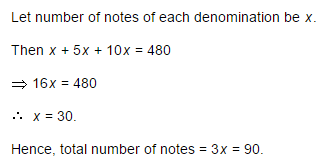Simplification And Approximation - Practice Test - Question 2

### There are two examinations rooms A and B. If 10 students are sent from A to B, then the number of students in each room is the same. If 20 candidates are sent from B to A, then the number of students in A is double the number of students in B. The number of students in room A is:

Detailed Solution for Simplification And Approximation - Practice Test - Question 2

Let the number of students in rooms A and B be x and y respectively.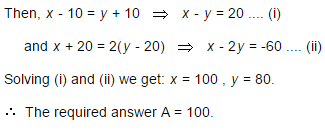Simplification And Approximation - Practice Test - Question 3

### The price of 10 chairs is equal to that of 4 tables. The price of 15 chairs and 2 tables together is Rs. 4000. The total price of 12 chairs and 3 tables is:

Detailed Solution for Simplification And Approximation - Practice Test - Question 3

Let the cost of a chair and that of a table be Rs. x and Rs. y respectively.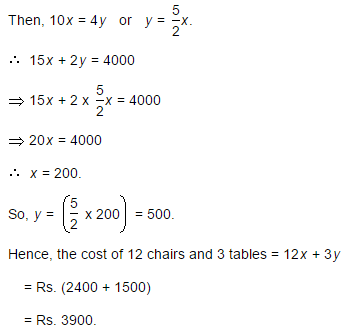Simplification And Approximation - Practice Test - Question 4

If a - b = 3 and a2 + b2 = 29, find the value of ab.

Detailed Solution for Simplification And Approximation - Practice Test - Question 4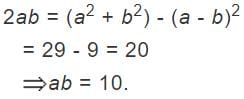Simplification And Approximation - Practice Test - Question 5

The price of 2 sarees and 4 shirts is Rs. 1600. With the same money one can buy 1 saree and 6 shirts. If one wants to buy 12 shirts, how much shall he have to pay ?

Detailed Solution for Simplification And Approximation - Practice Test - Question 5

Let the price of a saree and a shirt be Rs. x and Rs. y respectively.

Then, 2x + 4y = 1600 .... (i)

and x + 6y = 1600 .... (ii)

Divide equation (i) by 2, we get the below equation. => x + 2y = 800. --- (iii)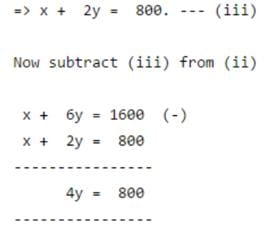Therefore, y = 200. Now apply value of y in (iii) => x + 2 x 200 = 800 => x + 400 = 800 Therefore x = 400

Solving (i) and (ii) we get x = 400, y = 200.

∴ Cost of 12 shirts = Rs. (12 x 200) = Rs. 2400.

## UPSC Prelims Paper 2 CSAT - Quant, Verbal & Decision Making

67 videos|50 docs|151 tests
 Use Code STAYHOME200 and get INR 200 additional OFF Use Coupon Code
Information about Simplification And Approximation - Practice Test Page
In this test you can find the Exam questions for Simplification And Approximation - Practice Test solved & explained in the simplest way possible. Besides giving Questions and answers for Simplification And Approximation - Practice Test, EduRev gives you an ample number of Online tests for practice

## UPSC Prelims Paper 2 CSAT - Quant, Verbal & Decision Making

67 videos|50 docs|151 tests